Search QuantityWare

# We are processing natural gas with a high hydrogen fraction, but not 100% hydrogen. What conversion groups can we use for such a gas?

As stated in the research paper from the DVGW: “Calculation of Compression Factors and Gas Law Deviation Factors Using the Modified SGERG-Equation SGERG-mod-H2”

“The AGA8 equation and the GERG-2008 equation generally agree better than ± 0.1% for the measured values for all data sets in the entire pressure range. It can thus be expected that these equations of state can be used for any desired H2 fractions without significantly affecting the underlying 0.1% uncertainty of the equations.”

Thus, BCG conversion groups that are configured to utilize AGA8 compression factor calculations may be utilized for natural gas / hydrogen mixtures of any ratio.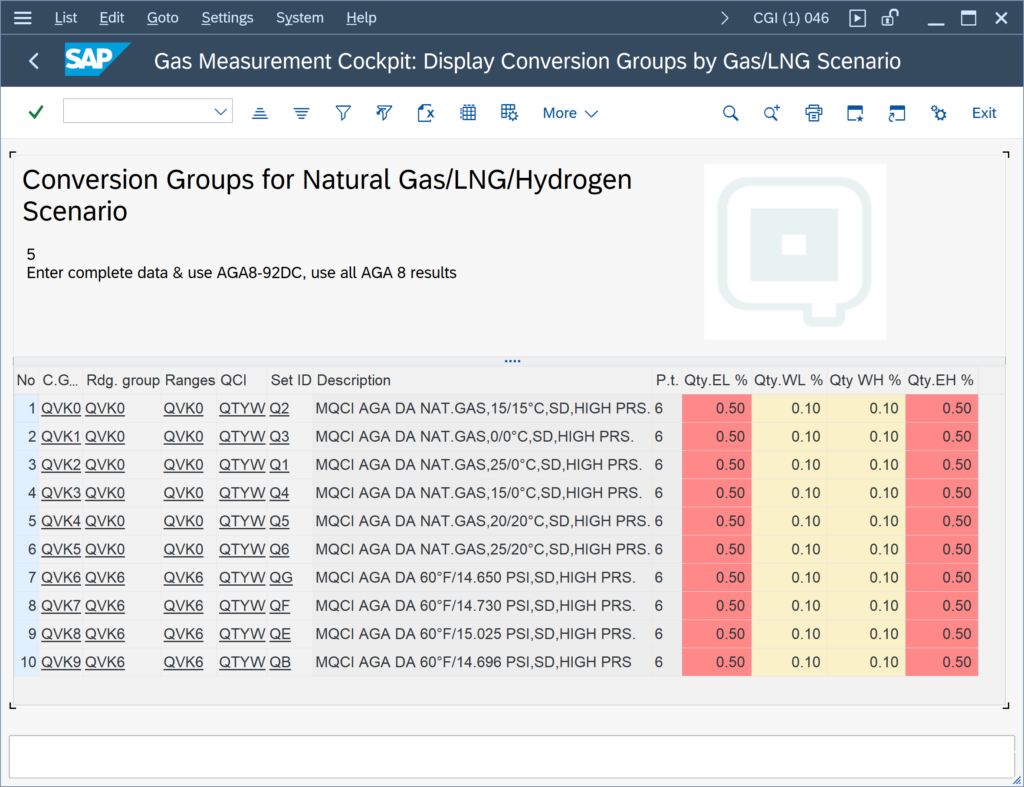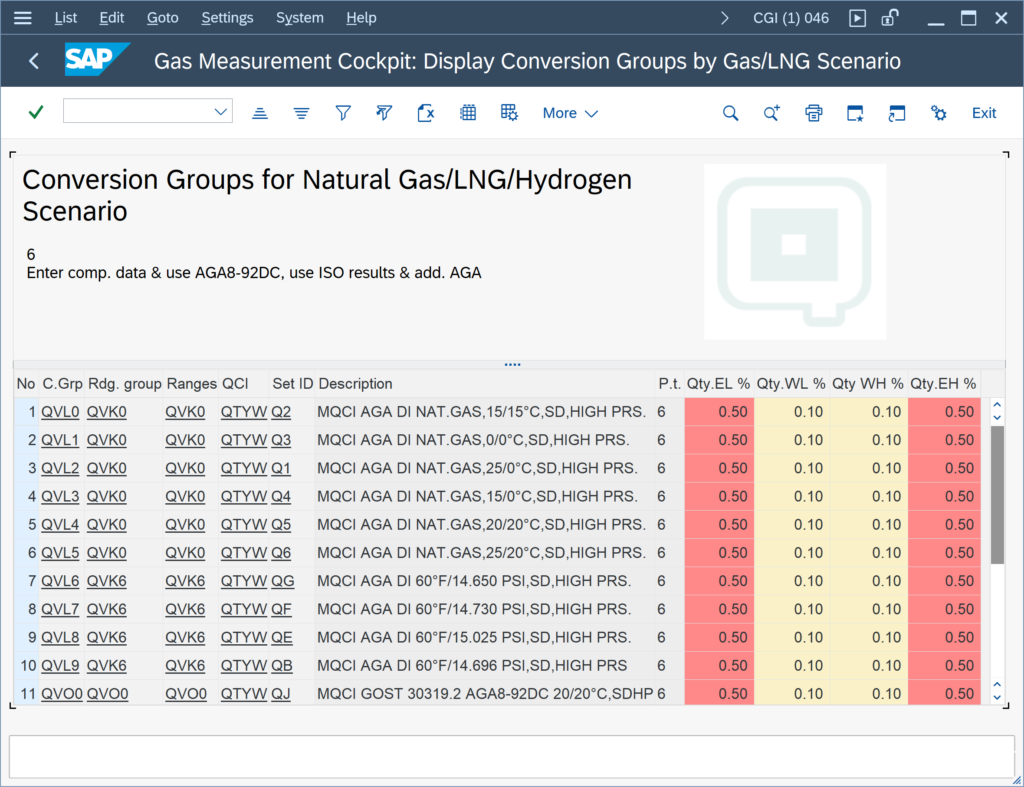Furthermore, the DVGW research paper states:

For the equation SGERG-88, for a hydrogen content of up to 10 mol-% and pressures up to 50 bar, an uncertainty of 0.1 % can be expected.

“The SGERG-88 equation (see section 3.1), which takes into account the calorific value, the density at standard conditions (in short standard density), the CO2 content, and the H2 content for the gas properties characterization, is mainly used in regional distribution networks, where the pressure is mostly below 50 bar, usually even below 25 bar.

Thus, for the conditions specified above, the following BCG template conversion groups may be utilized already: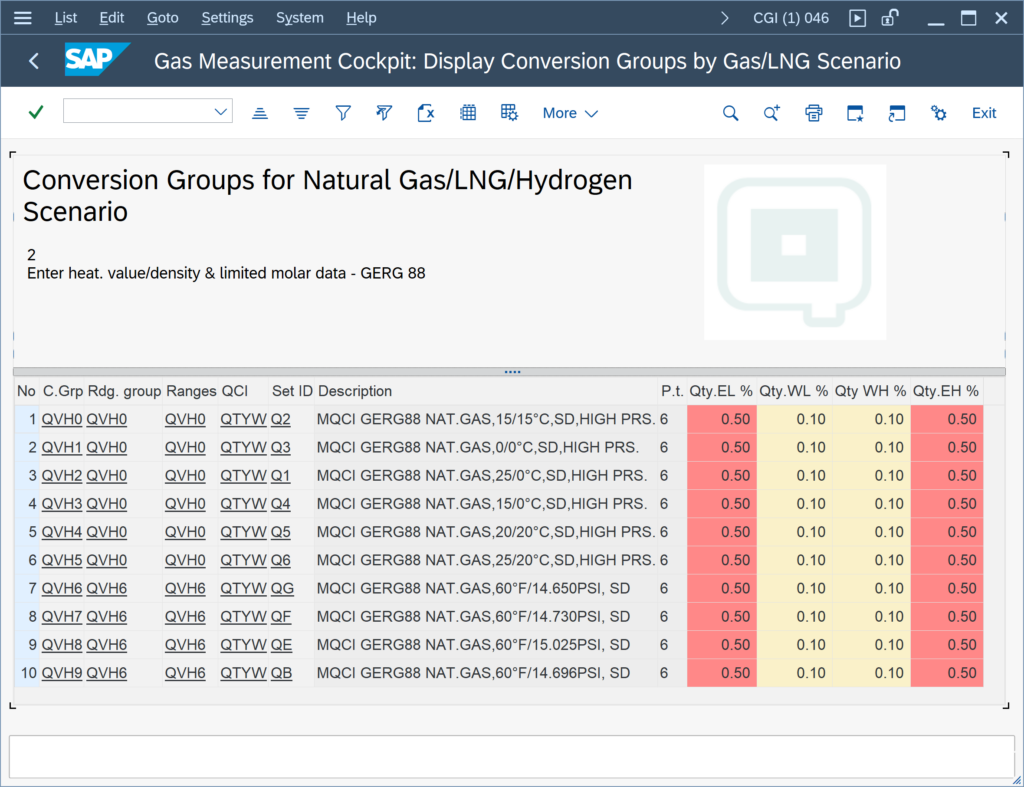With the next BCS CSP, QuantityWare will deliver a GERG-2008 implementation and related template conversion groups: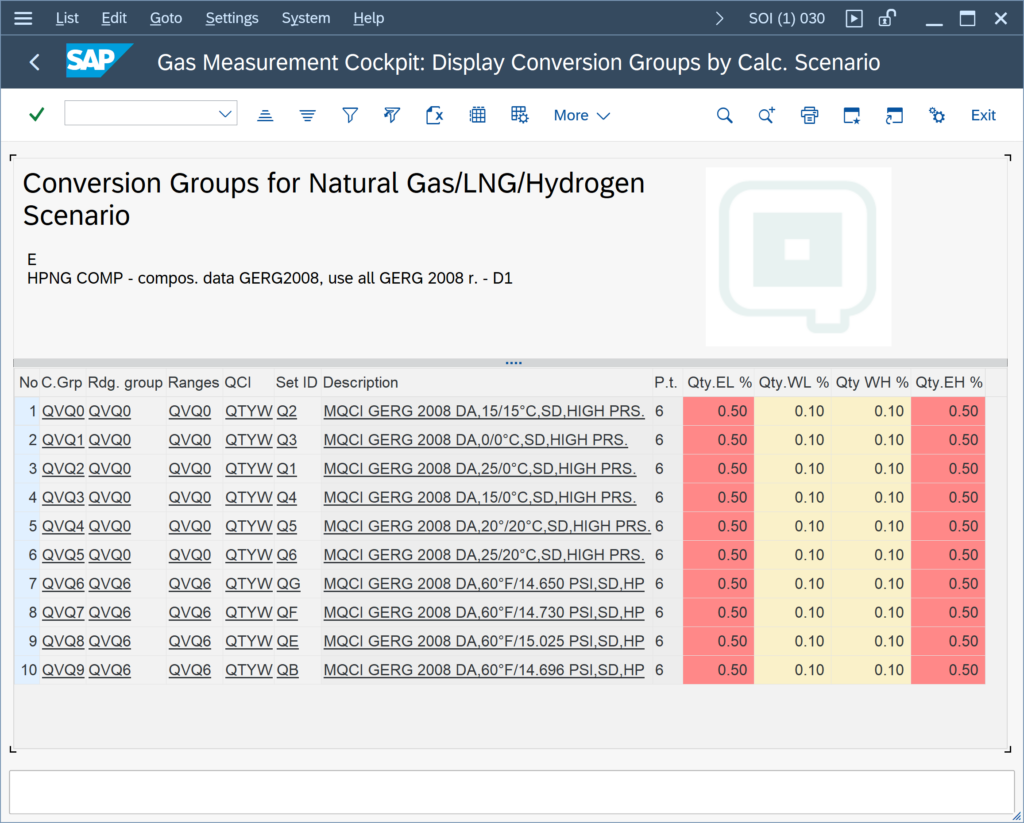Also, an implementation of the SGERG-mod-H2 approach (described in the DVGW research paper above), accompanied with new template conversion groups, will be delivered with the next CSP, such that the limitations given by the SGERG-88 equation are removed: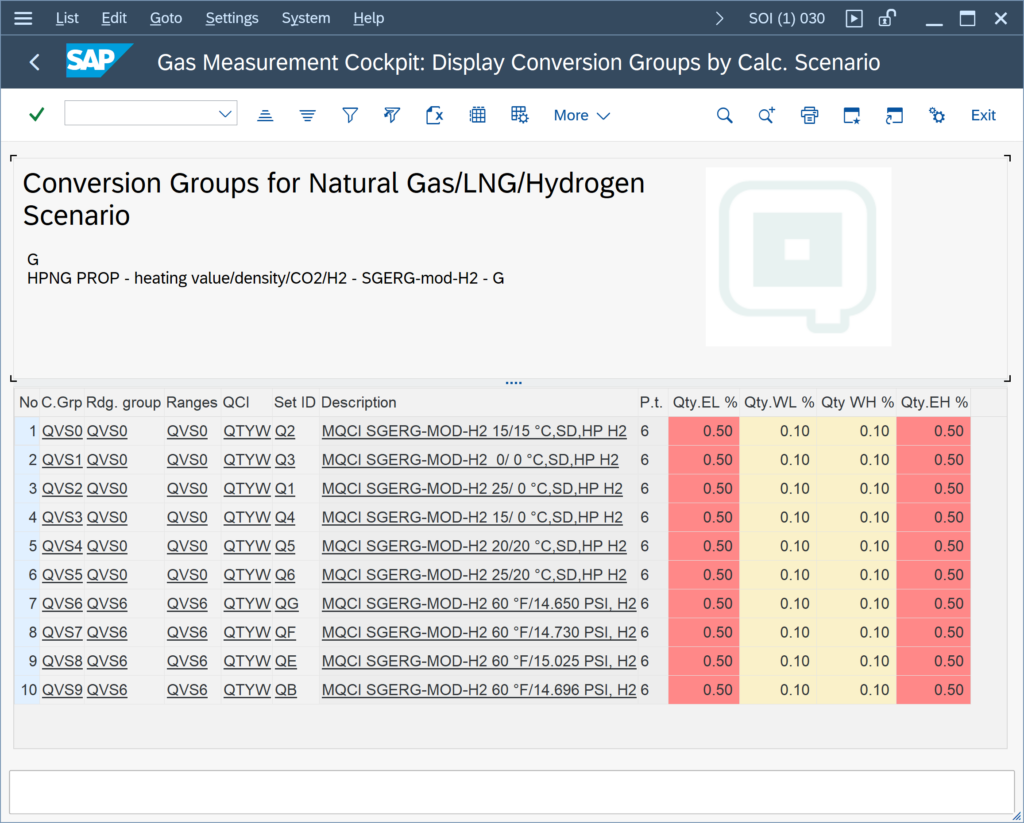Back to FAQs